Courses

# RD Sharma Solutions - Ex-2.2, Fractions, Class 7, Math Class 7 Notes | EduRev

## Class 7 : RD Sharma Solutions - Ex-2.2, Fractions, Class 7, Math Class 7 Notes | EduRev

The document RD Sharma Solutions - Ex-2.2, Fractions, Class 7, Math Class 7 Notes | EduRev is a part of the Class 7 Course RD Sharma Solutions for Class 7 Mathematics.
All you need of Class 7 at this link: Class 7

#### QUESTION 1:

Multiply: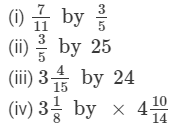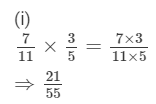(ii)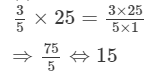(iii)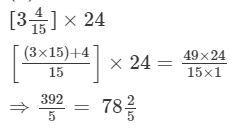(iv)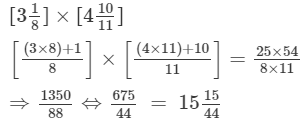#### QUESTION 2:

Find the product: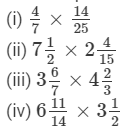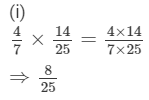(ii)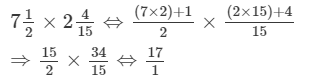(iii)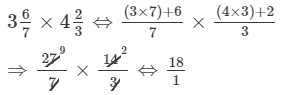(iv)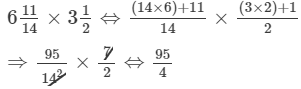#### QUESTION 3:

Simplify: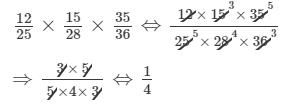(ii)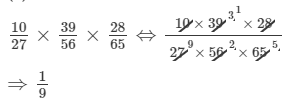(iii)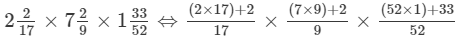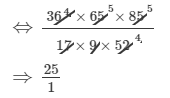#### QUESTION 4:

Find: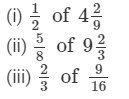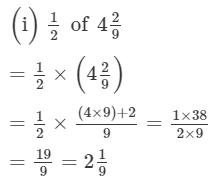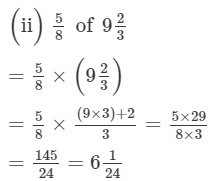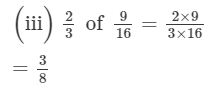#### QUESTION 5:

Which is greater?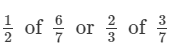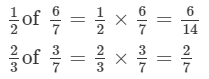convert  2/7 to its equivalent fraction with denominator as 14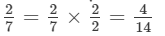we know 6>4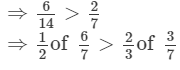#### QUESTION 6:

Find:

(i) 7/11 of Rs 330
(ii) 5/9 of 108 metres
(iii) 3/7 of 42 litres
(iv) 1/12 of an hour
(v) 5/of an year
(vi) 3/20 of a kg
(vii) 7/20 of a litre
(viii) 5/6 of a day
(ix) 2/of a week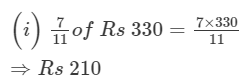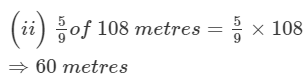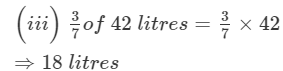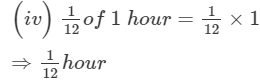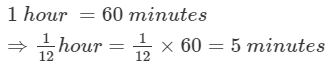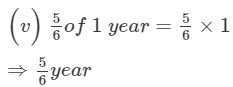1 year =12 months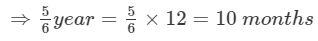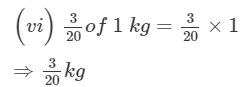1 kg =1000 g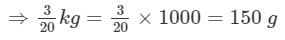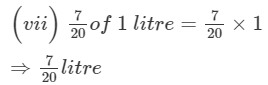1 l =1000 ml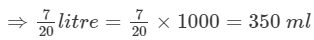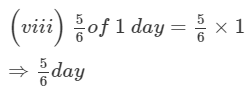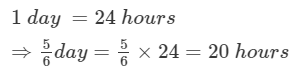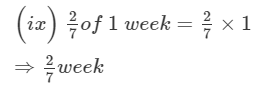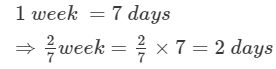#### Question 8:

Ravish reads 1/3 part of a book in 1 hour. How much part of the book will he read in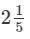houurs?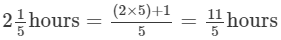In 1 hour Ravish reads 1/3 of the book

Part of book Ravish will read in 11/5 hours = Part read in 1 hour × 11/15

Part of book Ravish will read in 11/5 hours  =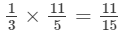#### Question 9:

Lipika reads a book for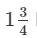hours everyday. She reads the entire book in 6 days. How many hours in all were required by her to read the book?

In one day, Lipika reads for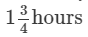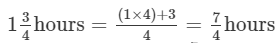Total hours required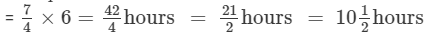#### Question 10:

Find the area of a rectangular park which is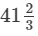m long and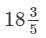m broad.

Length of rectangular park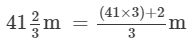⇒Length of rectangular park  = 125/3 m

Width of rectangular park =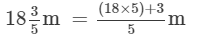⇒Width of rectangular park  = 93/5 m

Area of rectangular park = Length of rectangular park × Width of rectangular park

Area of rectangular park =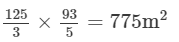#### Question 11:

If milk is available at Rs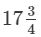per litre, find the cost of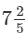litres of milk.

Cost of 1 litre milk = Rs.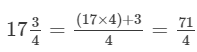⇒Cost of 1 litre milk =Rs. 71/4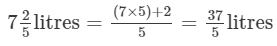Cost of 37/5litres milk = Cost of 1 litre milk x 37/5

Cost of 37/5litres milk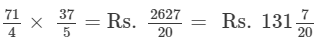#### Question 12:

Sharda can walk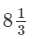km813 km in one hour. How much distance will she cover in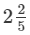hours?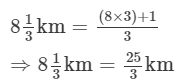Distance covered by ​Sharda in 1 hour =  25/3 km.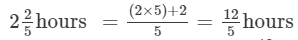Distance covered by Sharda in 12/5hours = Distance covered in 1 hour × (12/50

⇒⇒Distance covered by Sharda in 12/5 hours =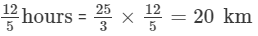#### Question 13:

A sugar bag contains 30 kg of sugar. After consuming 2/3 of it, how much sugar is left in the bag?

Total amount of sugar in the bag = 30 kg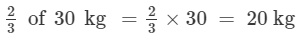⇒Amount of sugar consumed = 20 kg

Sugar left in the bag = Total amount of sugar in the bag − Amount of sugar consumed

Sugar left in the bag = 30−20 =10 kg

#### Question 14:

Each side of a square is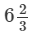m long. Find its area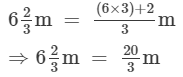Area of square=Side × Side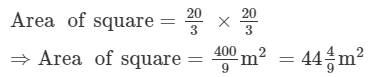#### Question 15:

There are 45 students in a class and 3/5 of them are boys. How many girls are there in the class?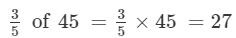Therefore, 27 students are boys.

Number of girls=Total number of students in the class − Number of boys

Number of girls=45−27=18

Therefore, 18 students are girls.

Offer running on EduRev: Apply code STAYHOME200 to get INR 200 off on our premium plan EduRev Infinity!

97 docs

,

,

,

,

,

,

,

,

,

,

,

,

,

,

,

,

,

,

,

,

,

,

,

,

,

,

,

,

,

,

;